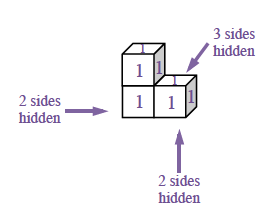Home > CC2 > Chapter 9 > Lesson 9.2.1 > Problem9-56

9-56.
1. Find the volume and surface area of each shape below. Assume that the edge of each cube measures 1". Homework Help ✎

1.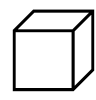2.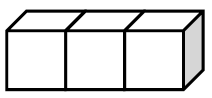3.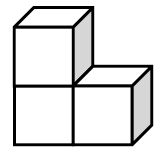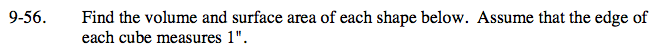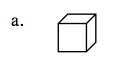Each of these objects are three-dimensional and have length, width, and depth.

Volume is the number of 1-inch cubes needed to fill a three dimensional object.

The surface area of an object is the total area of all its surfaces.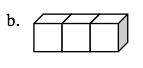See part (a).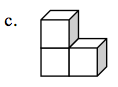Including the hidden sides, there are 14 total square sides each with area 1 sq in. There are 3 cubes so the volume
is 3 in³.